# RD Sharma Solutions for Class 8 Chapter 3 - Squares and Square Roots Exercise 3.5

Students can download the RD Sharma Solutions for the Exercise 3.5 of Class 8 Maths Chapter 3, Squares and Square Roots from the link accessible here. BYJU’S masters have solved the questions present in Exercise 3.5 in Maths, and this will help students in solving this exercise without any dilemmas. By practising regularly, the students can obtain high scores in the Class 8 exams by using the RD Sharma Solutions. This exercise helps students to understand the concept of finding the square root of a perfect square by the long-division method.

By practising the RD Sharma Class 8 Solutions students will be able to understand the concepts clearly which in turn helps in gaining confidence, which plays a vital role in their examinations.

## Download the pdf of RD Sharma For Class 8 Maths Chapter 3 Squares and Square Roots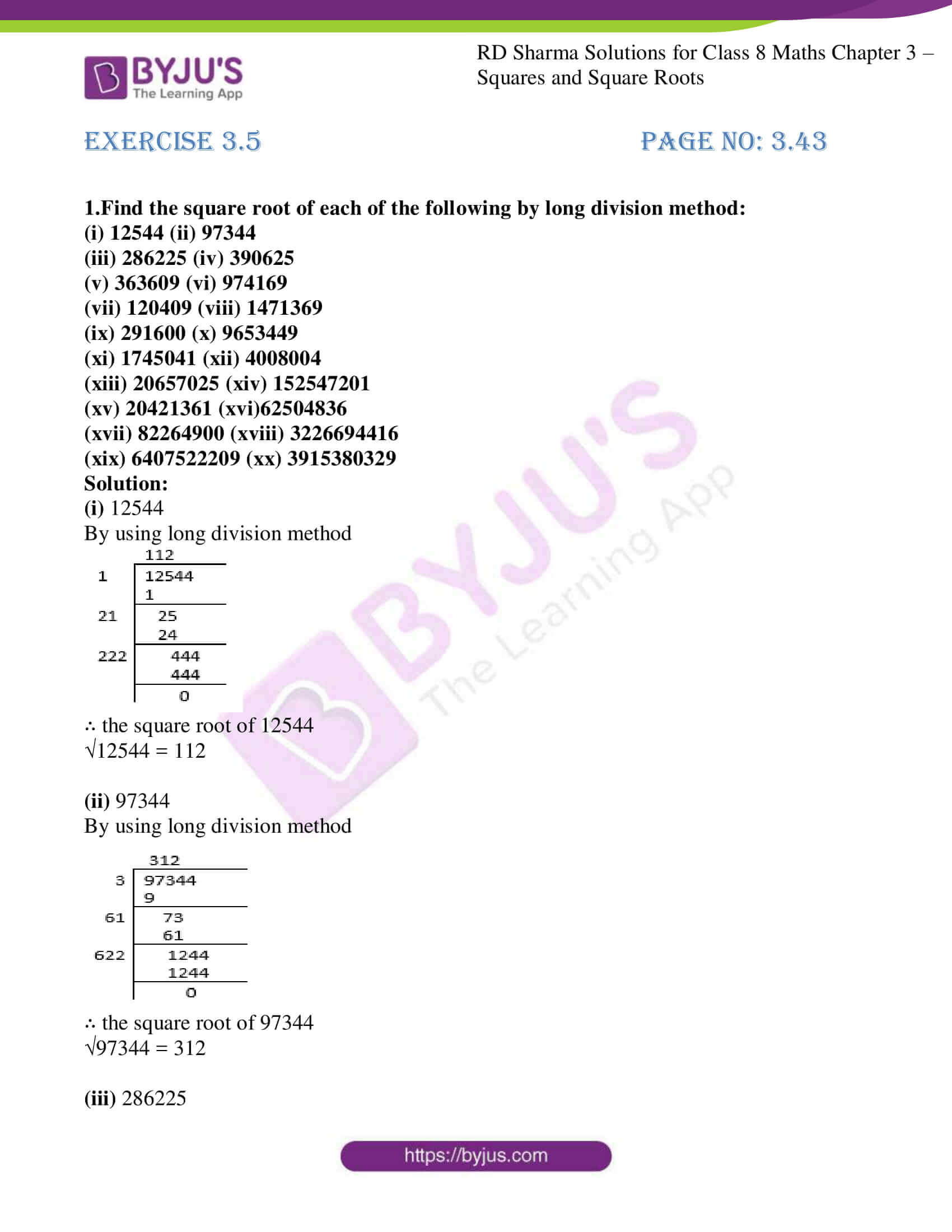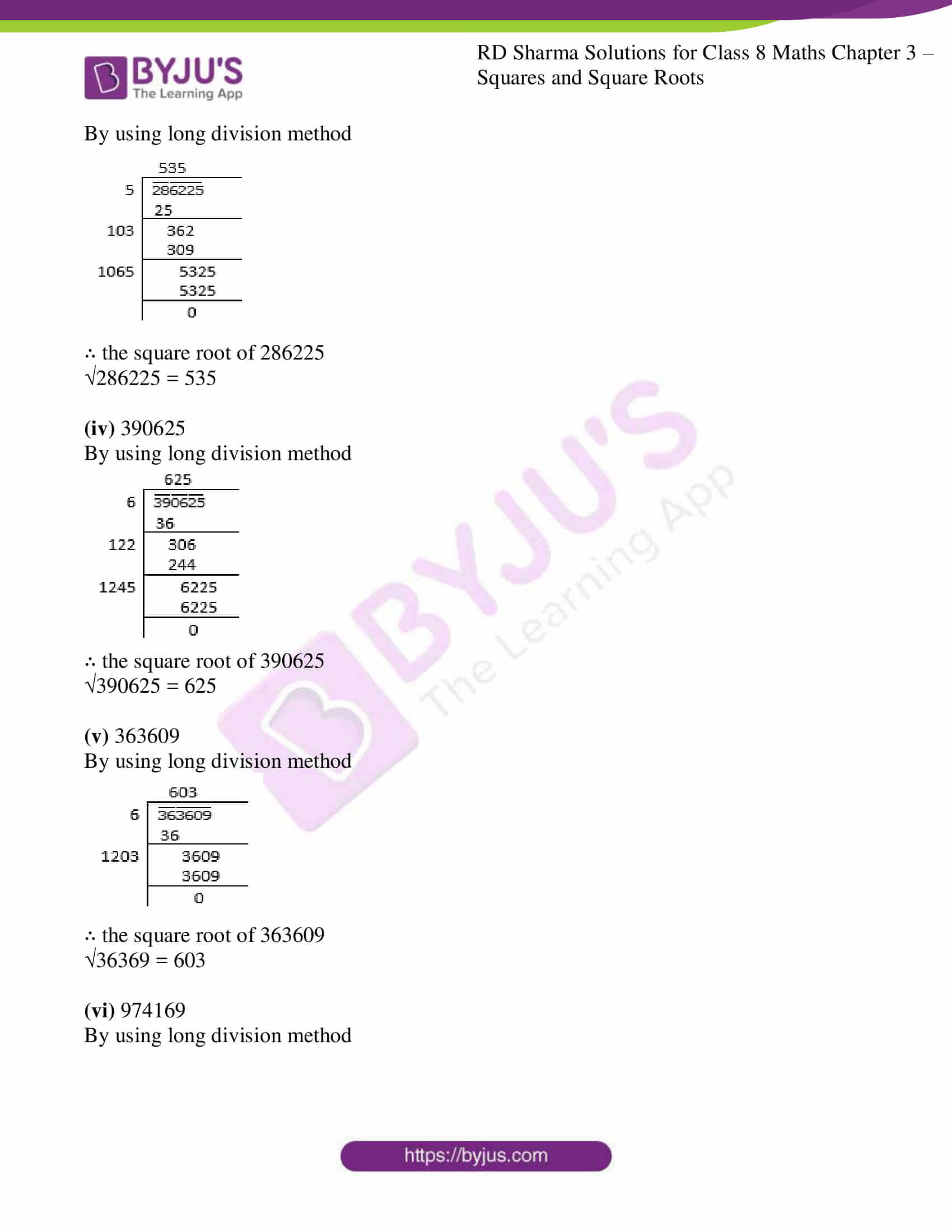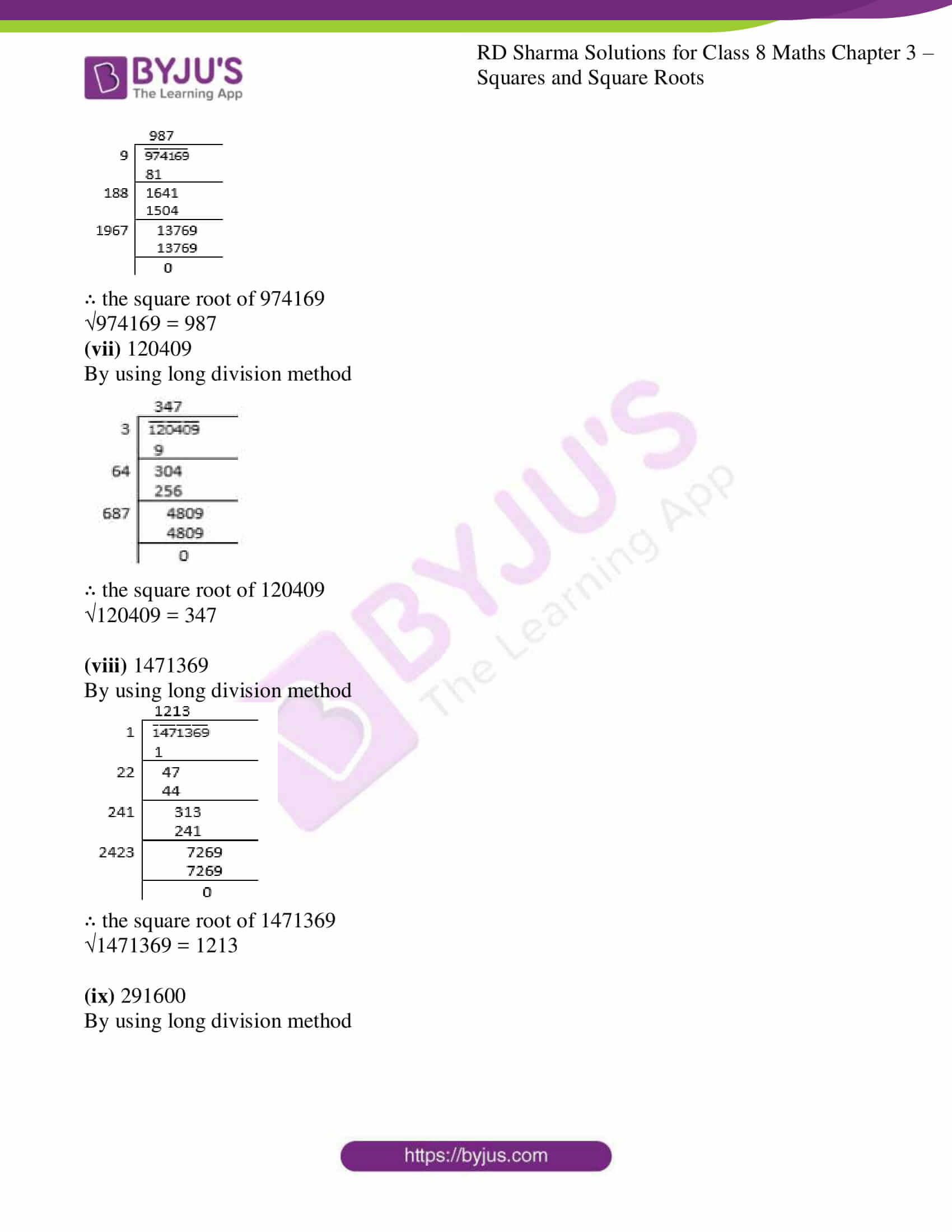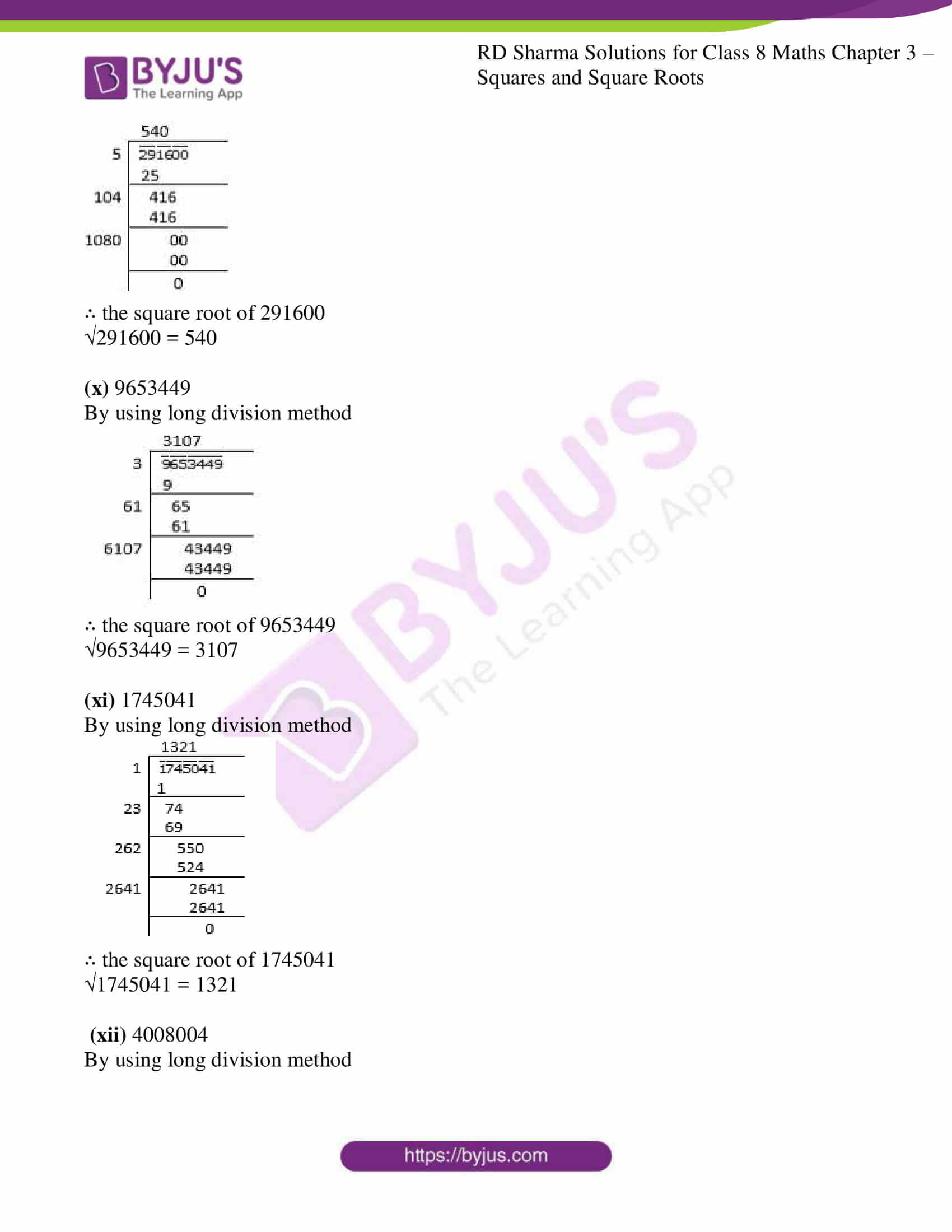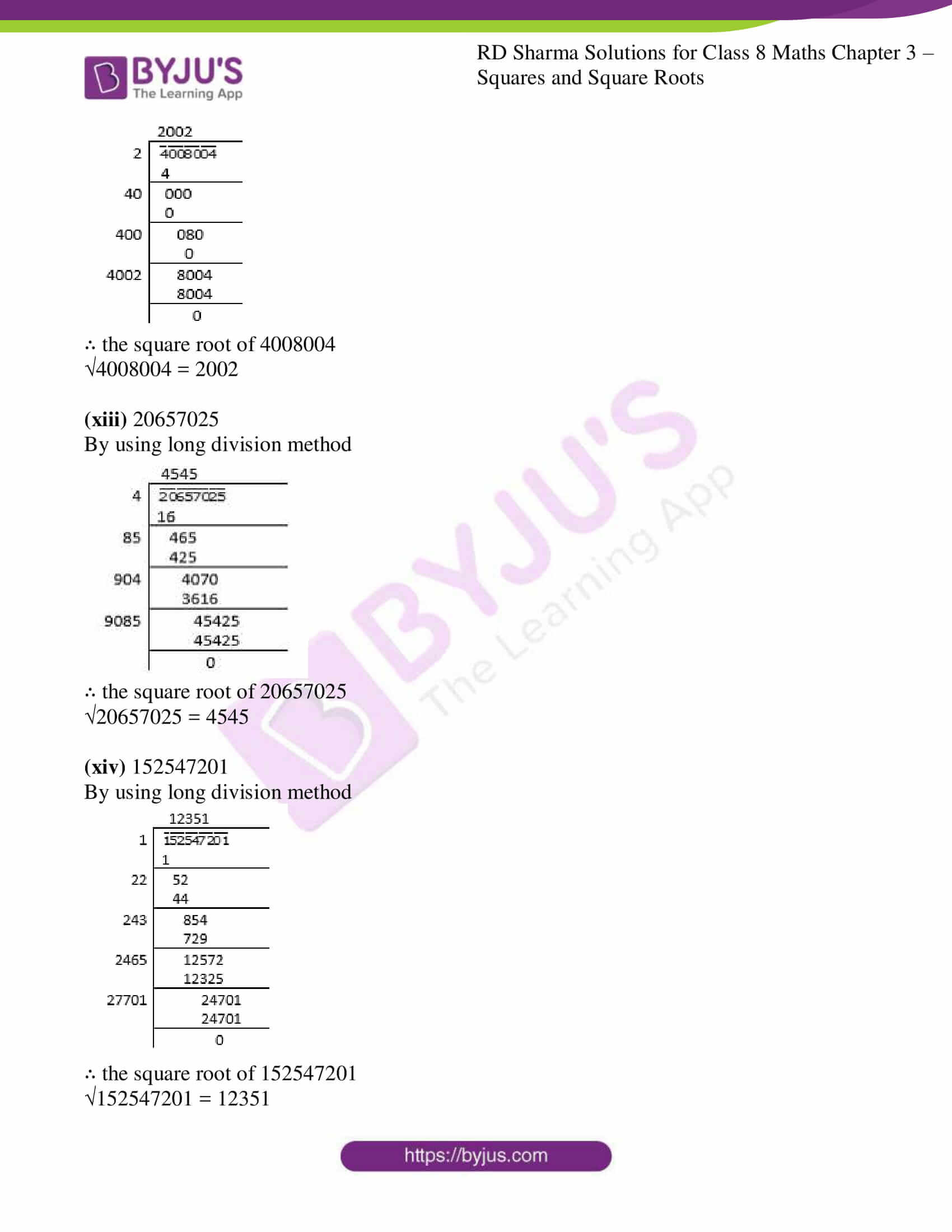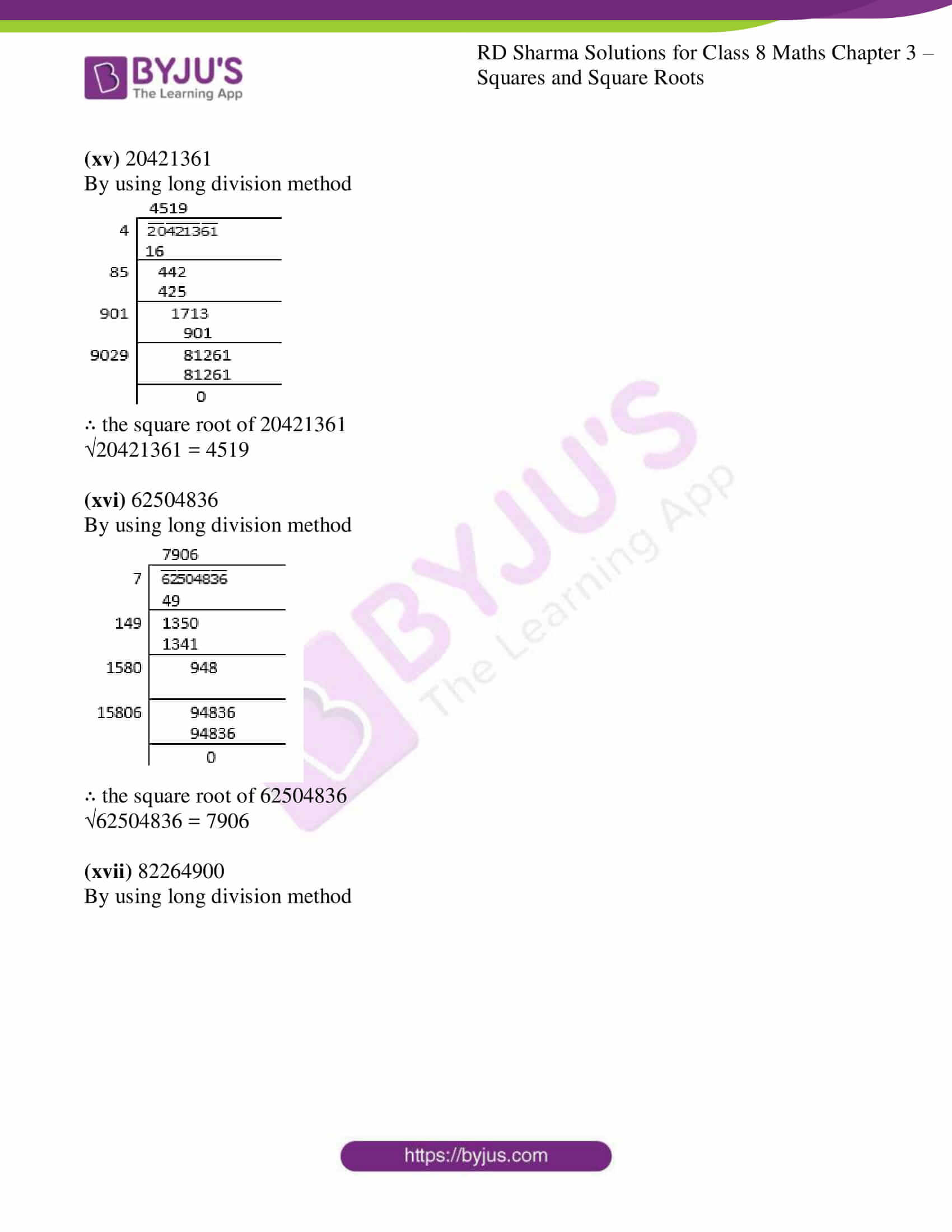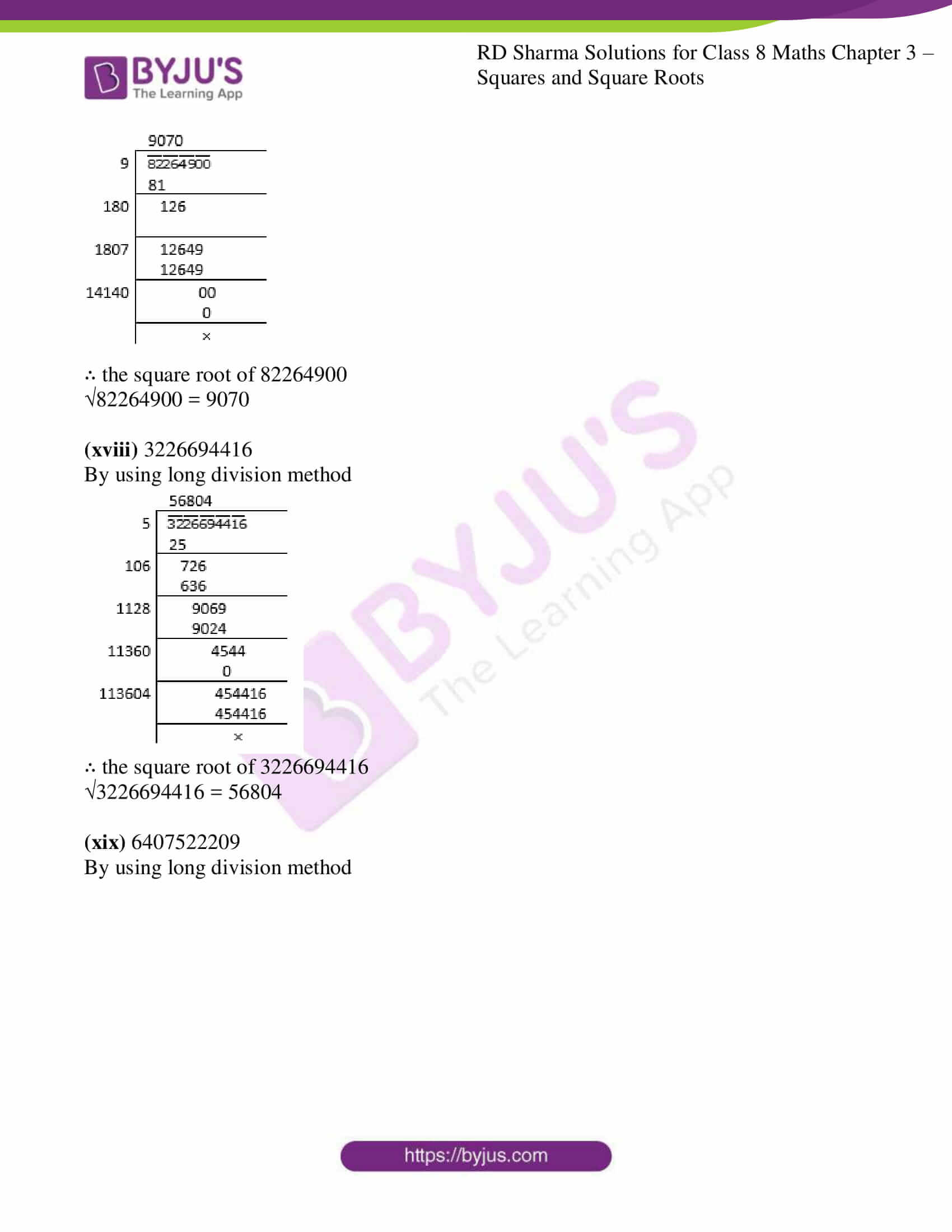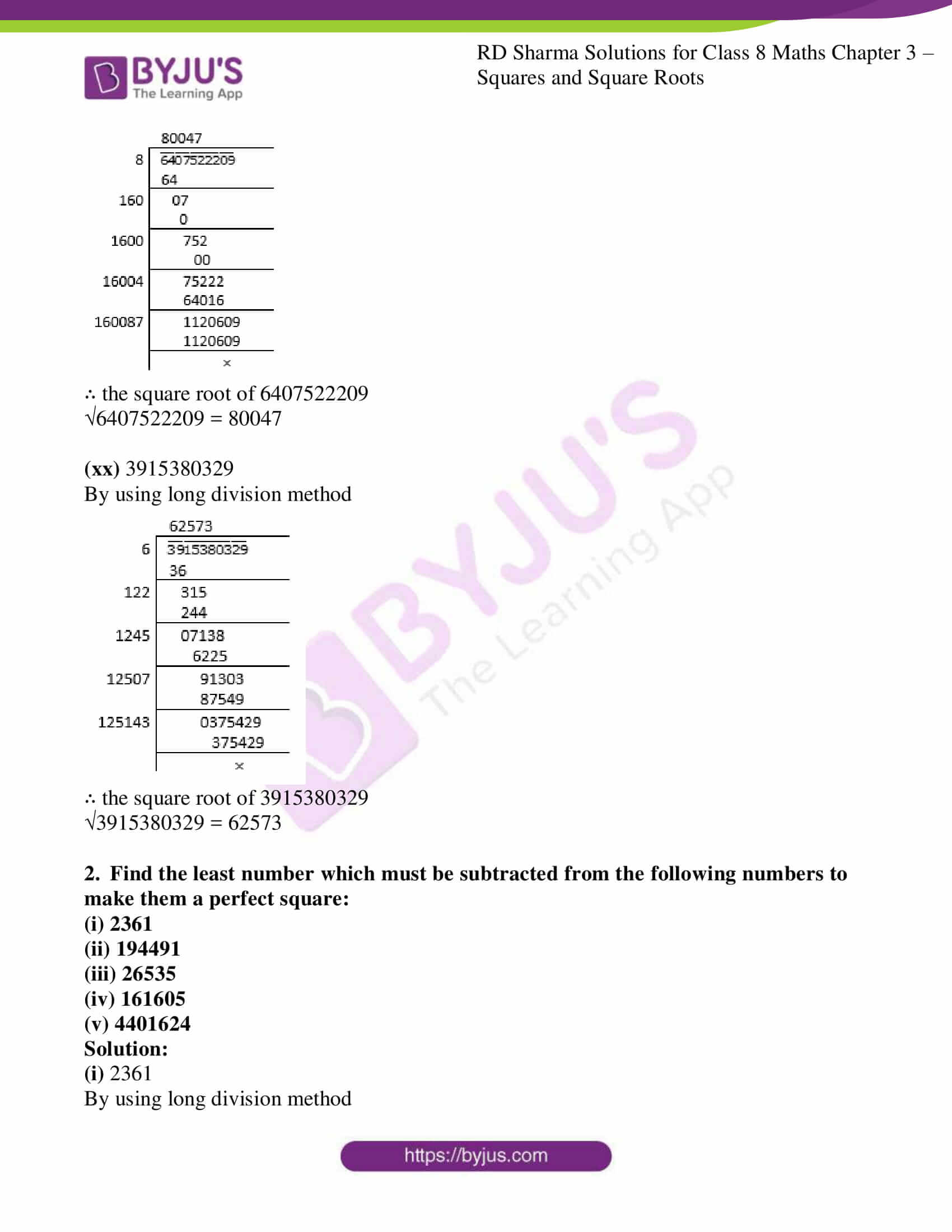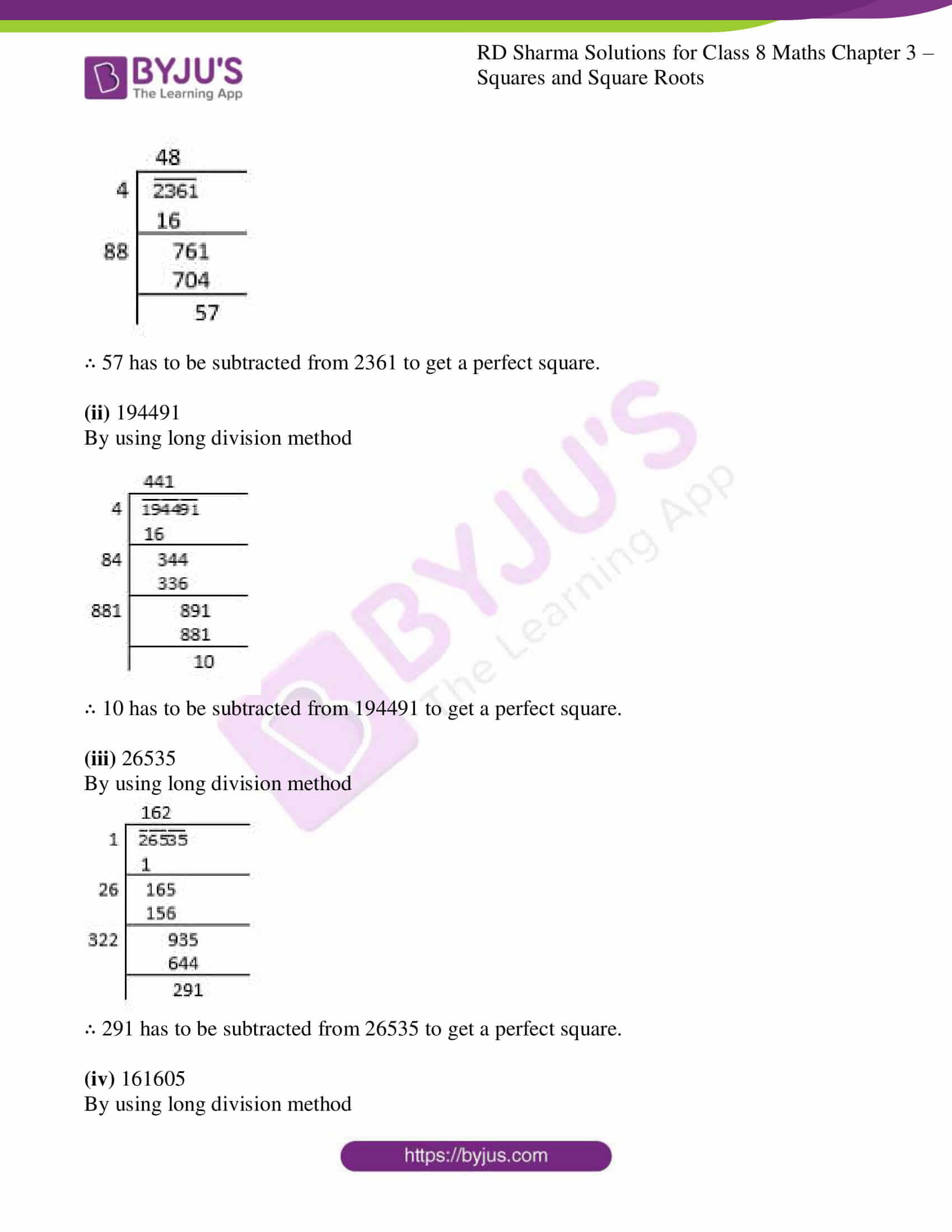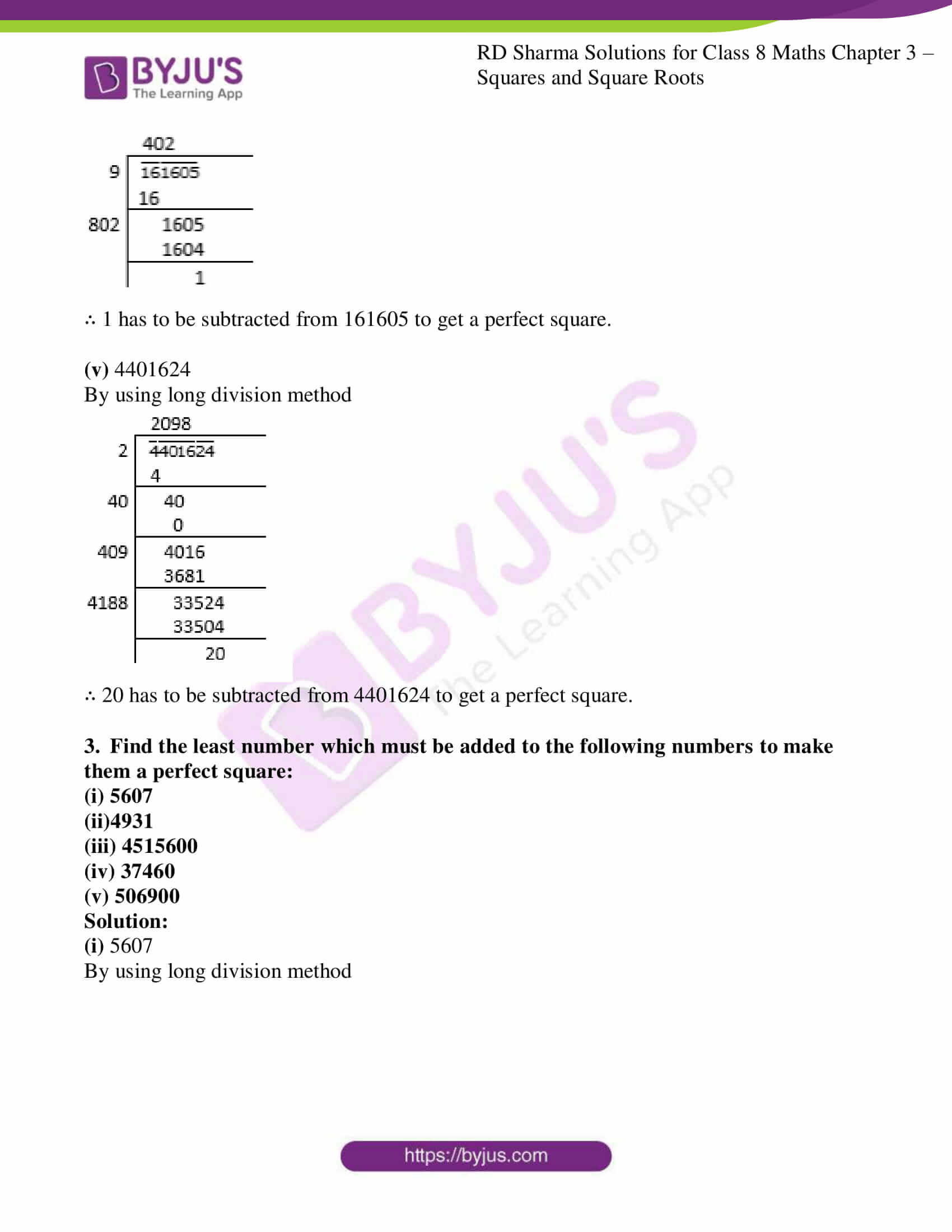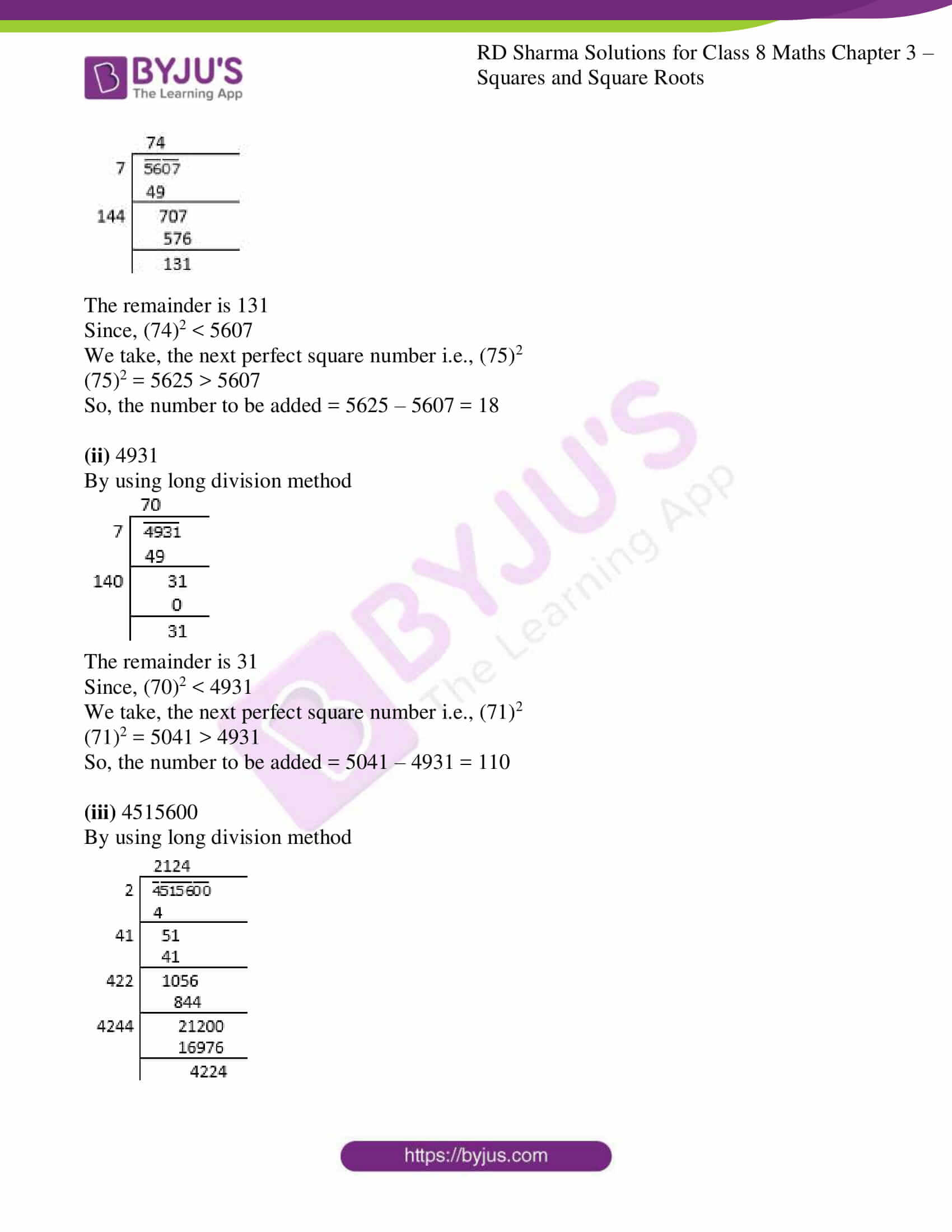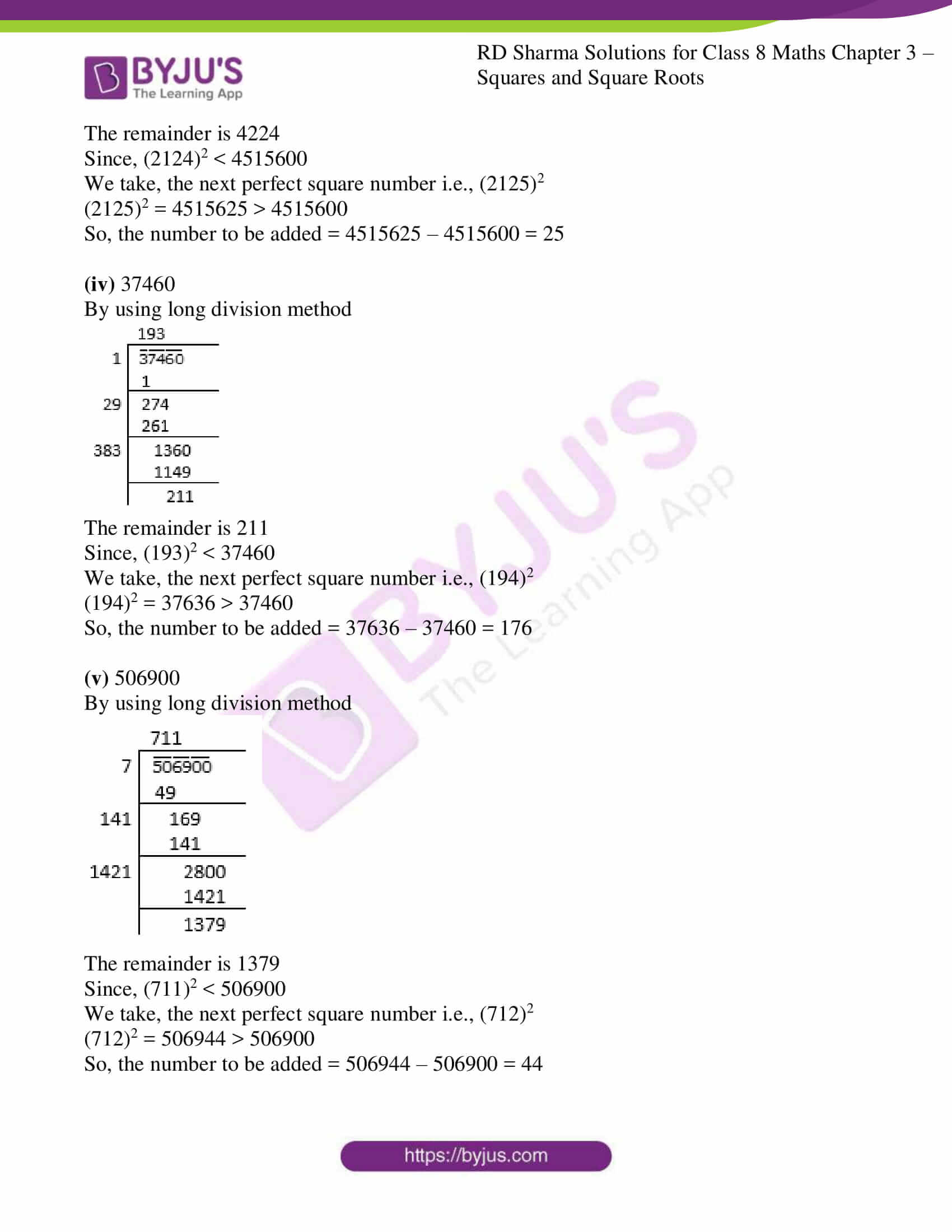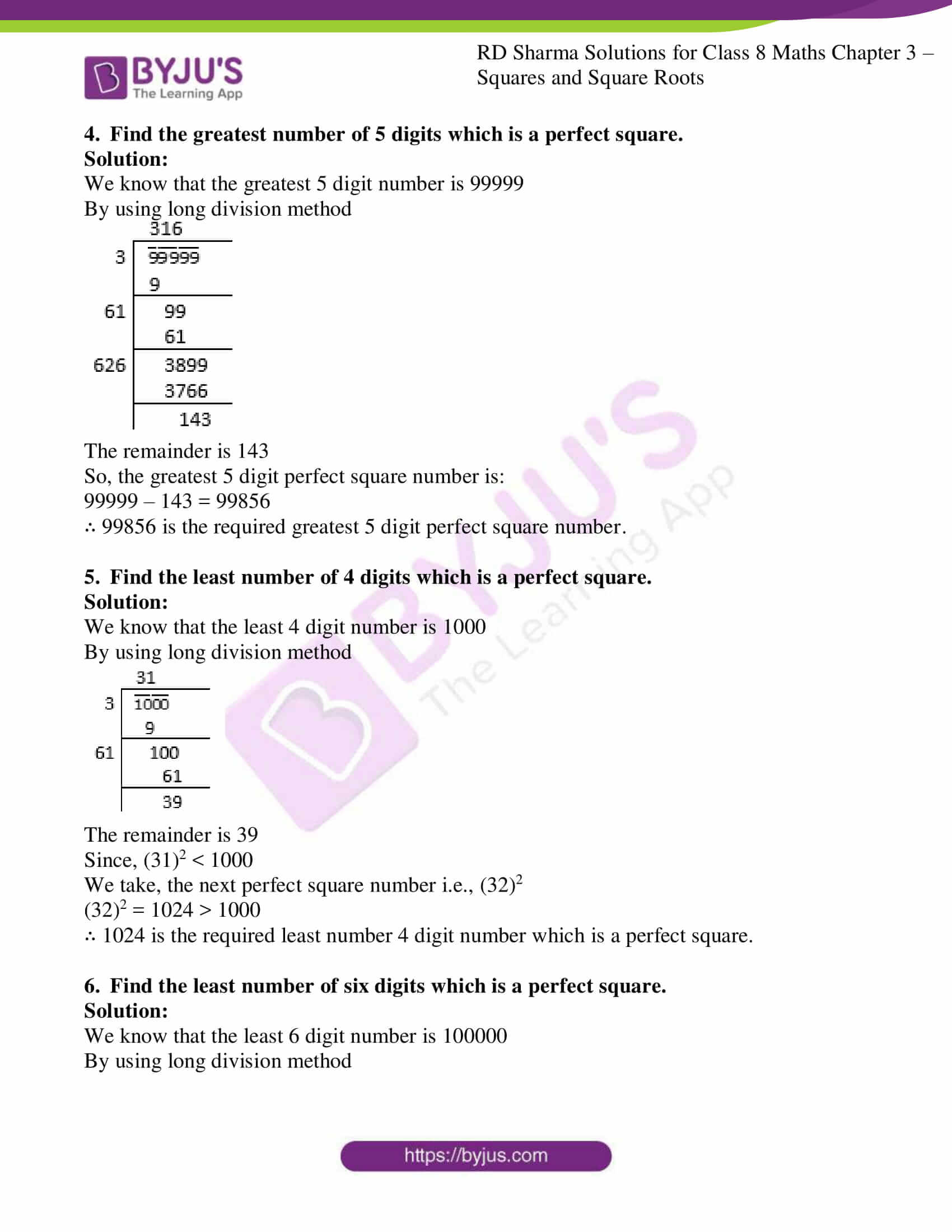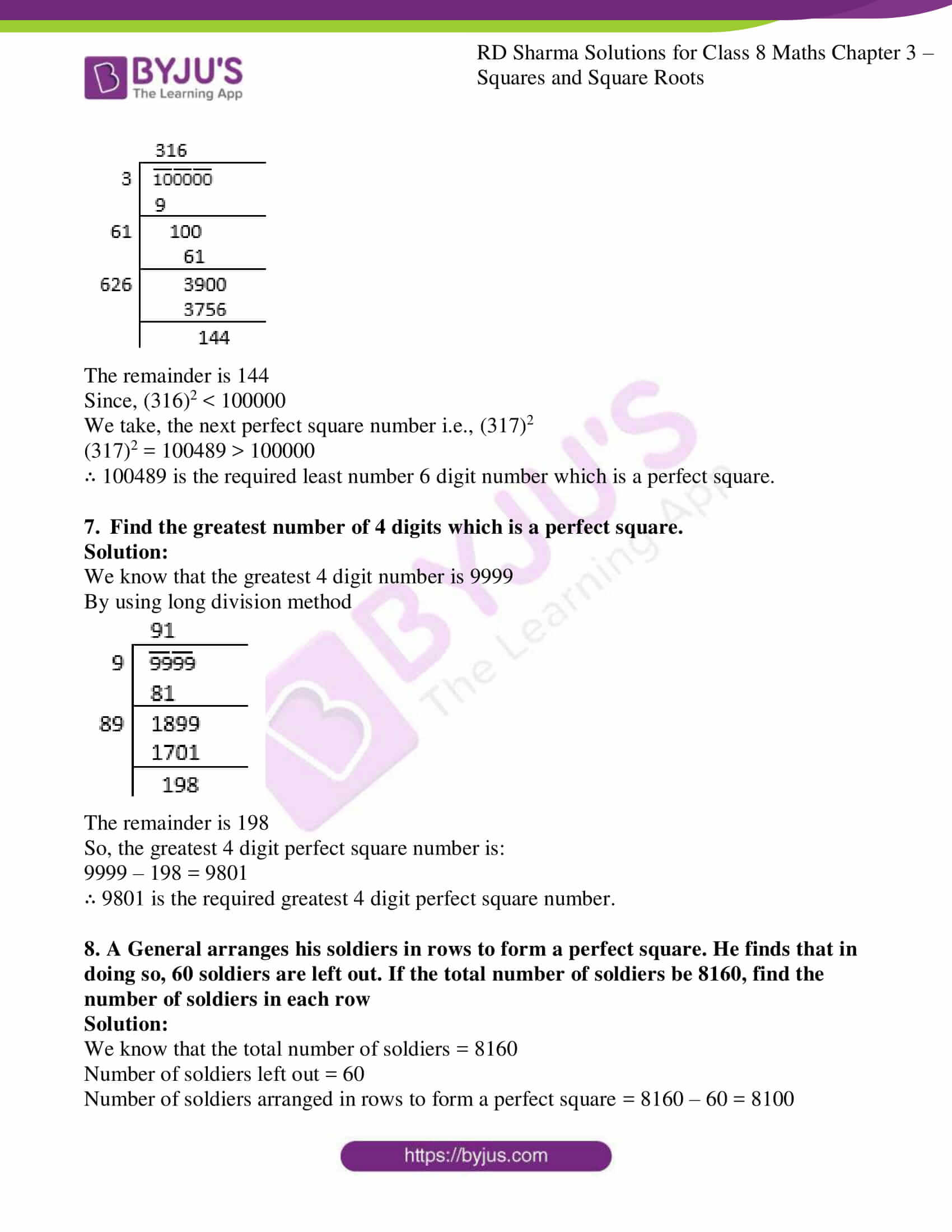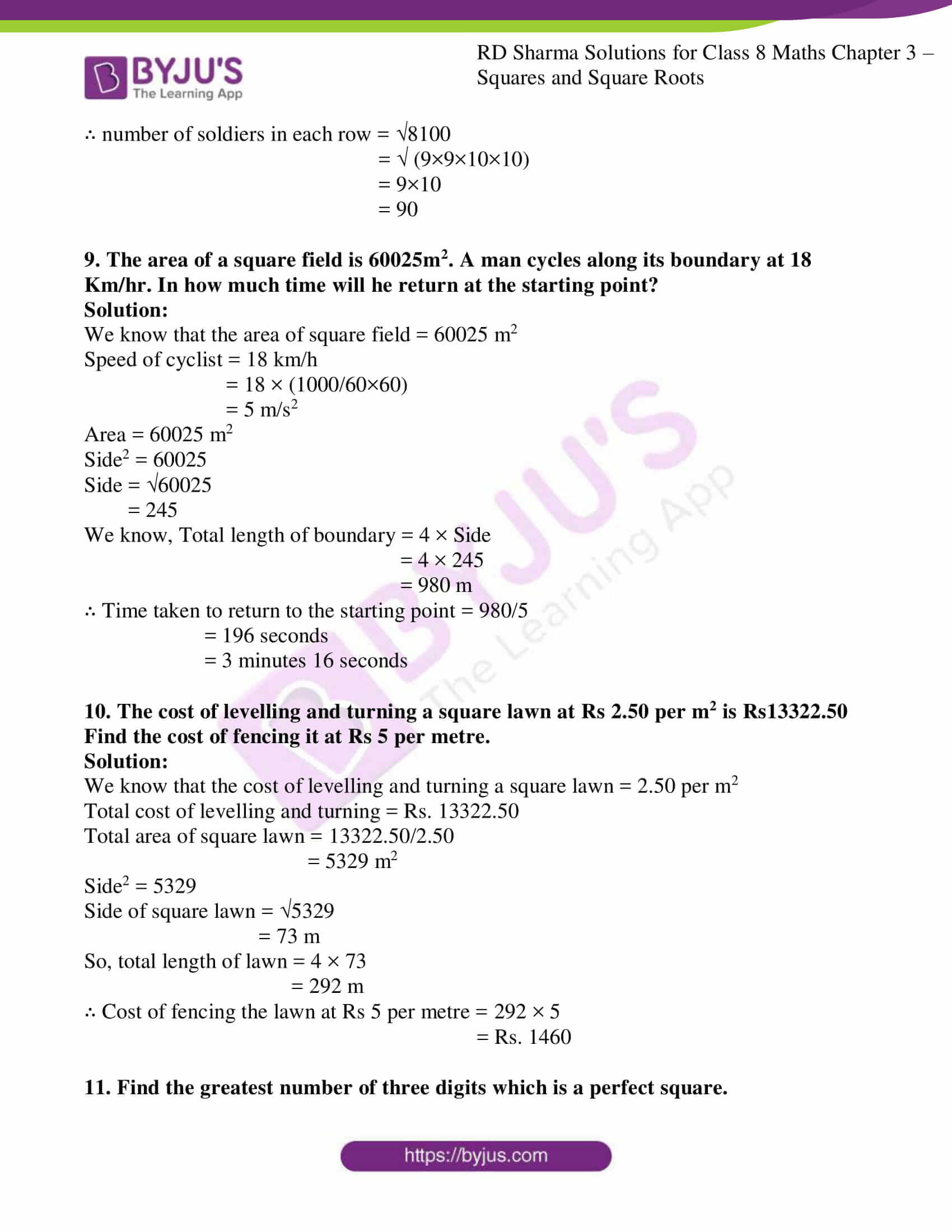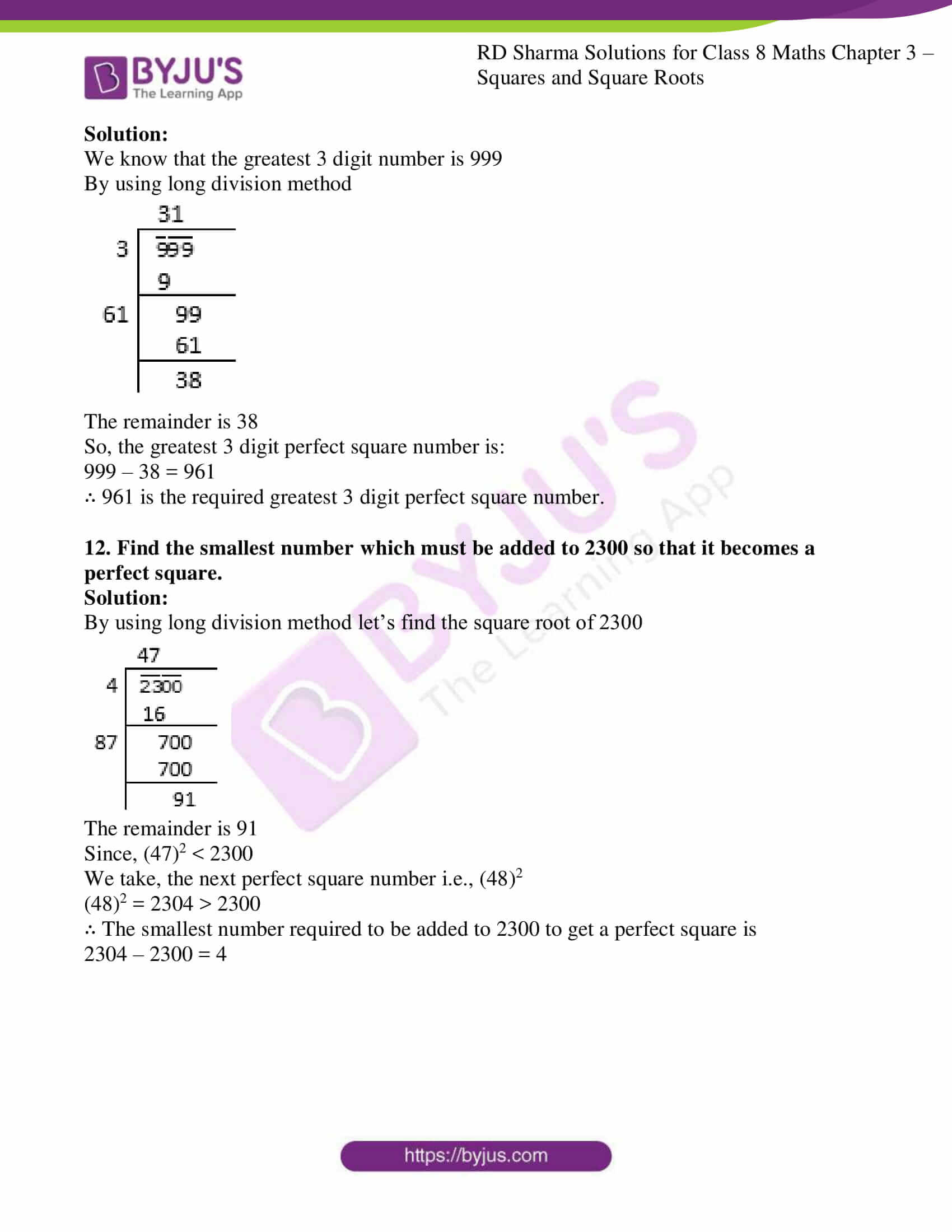### Access Answers to RD Sharma Solutions for Class 8 Maths Exercise 3.5 Chapter 3 Squares and Square Roots

1.Find the square root of each of the following by long division method:
(i) 12544 (ii) 97344
(iii) 286225 (iv) 390625
(v) 363609 (vi) 974169
(vii) 120409 (viii) 1471369
(ix) 291600 (x) 9653449
(xi) 1745041 (xii) 4008004
(xiii) 20657025 (xiv) 152547201
(xv) 20421361 (xvi)62504836
(xvii) 82264900 (xviii) 3226694416
(xix) 6407522209 (xx) 3915380329

Solution:

(i) 12544

By using long division method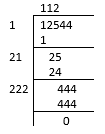∴ the square root of 12544

√12544 = 112

(ii) 97344

By using long division method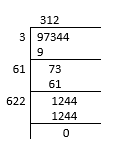∴ the square root of 97344

√97344 = 312

(iii)
286225

By using long division method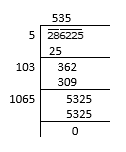∴ the square root of 286225

√286225 = 535

(iv) 390625

By using long division method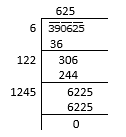∴ the square root of 390625

√390625 = 625

(v)
363609

By using long division method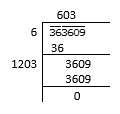∴ the square root of 363609

√36369 = 603

(vi) 974169

By using long division method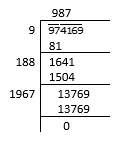∴ the square root of 974169

√974169 = 987

(vii) 120409

By using long division method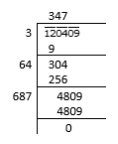∴ the square root of 120409

√120409 = 347

(viii) 1471369

By using long division method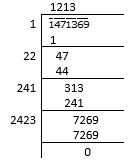∴ the square root of 1471369

√1471369 = 1213

(ix)
291600

By using long division method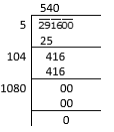∴ the square root of 291600

√291600 = 540

(x) 9653449

By using long division method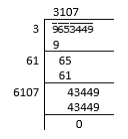∴ the square root of 9653449

√9653449 = 3107

(xi)
1745041

By using long division method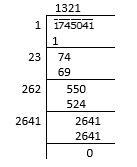∴ the square root of 1745041

√1745041 = 1321

(xii) 4008004

By using long division method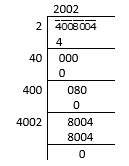∴ the square root of 4008004

√4008004 = 2002

(xiii)
20657025

By using long division method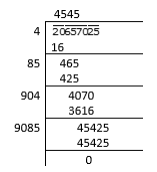∴ the square root of 20657025

√20657025 = 4545

(xiv) 152547201

By using long division method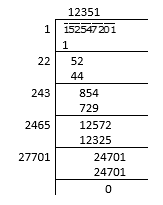∴ the square root of 152547201

√152547201 = 12351

(xv)
20421361

By using long division method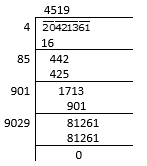∴ the square root of 20421361

√20421361 = 4519

(xvi) 62504836

By using long division method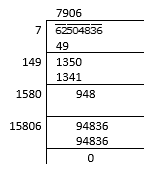∴ the square root of 62504836

√62504836 = 7906

(xvii)
82264900

By using long division method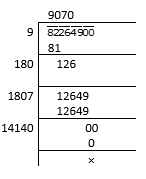∴ the square root of 82264900

√82264900 = 9070

(xviii) 3226694416

By using long division method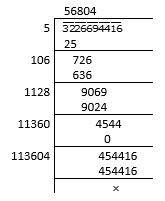∴ the square root of 3226694416

√3226694416 = 56804

(xix)
6407522209

By using long division method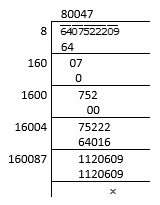∴ the square root of 6407522209

√6407522209 = 80047

(xx) 3915380329

By using long division method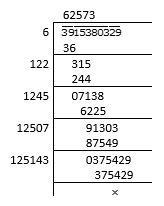∴ the square root of 3915380329

√3915380329 = 62573

2. Find the least number which must be subtracted from the following numbers to make them a perfect square:
(i) 2361
(ii) 194491
(iii) 26535
(iv) 161605
(v) 4401624

Solution:

(i) 2361

By using long division method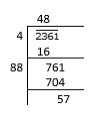∴ 57 has to be subtracted from 2361 to get a perfect square.

(ii)
194491

By using long division method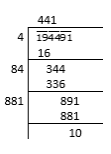∴ 10 has to be subtracted from 194491 to get a perfect square.

(iii)
26535

By using long division method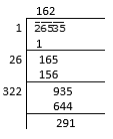∴ 291 has to be subtracted from 26535 to get a perfect square.

(iv)
161605

By using long division method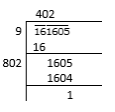∴ 1 has to be subtracted from 161605 to get a perfect square.

(v)
4401624

By using long division method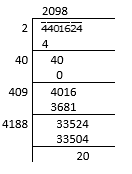∴ 20 has to be subtracted from 4401624 to get a perfect square.

3. Find the least number which must be added to the following numbers to make them a perfect square:
(i) 5607
(ii)4931
(iii) 4515600
(iv) 37460
(v) 506900

Solution:

(i) 5607

By using long division method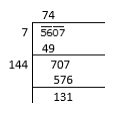The remainder is 131

Since, (74)2 < 5607

We take, the next perfect square number i.e., (75)2

(75)2 = 5625 > 5607

So, the number to be added = 5625 – 5607 = 18

(ii)
4931

By using long division method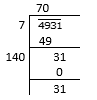The remainder is 31

Since, (70)2 < 4931

We take, the next perfect square number i.e., (71)2

(71)2 = 5041 > 4931

So, the number to be added = 5041 – 4931 = 110

(iii)
4515600

By using long division method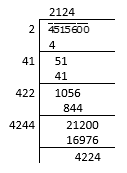The remainder is 4224

Since, (2124)2 < 4515600

We take, the next perfect square number i.e., (2125)2

(2125)2 = 4515625 > 4515600

So, the number to be added = 4515625 – 4515600 = 25

(iv)
37460

By using long division method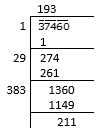The remainder is 211

Since, (193)2 < 37460

We take, the next perfect square number i.e., (194)2

(194)2 = 37636 > 37460

So, the number to be added = 37636 – 37460 = 176

(v)
506900

By using long division method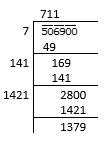The remainder is 1379

Since, (711)2 < 506900

We take, the next perfect square number i.e., (712)2

(712)2 = 506944 > 506900

So, the number to be added = 506944 – 506900 = 44

4. Find the greatest number of 5 digits which is a perfect square.

Solution:

We know that the greatest 5 digit number is 99999

By using long division method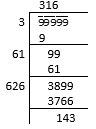The remainder is 143

So, the greatest 5 digit perfect square number is:

99999 – 143 = 99856

∴ 99856 is the required greatest 5 digit perfect square number.

5. Find the least number of 4 digits which is a perfect square.

Solution:

We know that the least 4 digit number is 1000

By using long division method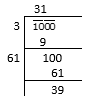The remainder is 39

Since, (31)2 < 1000

We take, the next perfect square number i.e., (32)2

(32)2 = 1024 > 1000

∴ 1024 is the required least number 4 digit number which is a perfect square.

6. Find the least number of six digits which is a perfect square.

Solution:

We know that the least 6 digit number is 100000

By using long division method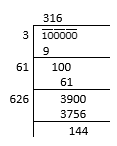The remainder is 144

Since, (316)2 < 100000

We take, the next perfect square number i.e., (317)2

(317)2 = 100489 > 100000

∴ 100489 is the required least number 6 digit number which is a perfect square.

7. Find the greatest number of 4 digits which is a perfect square.

Solution:

We know that the greatest 4 digit number is 9999

By using long division method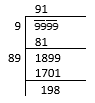The remainder is 198

So, the greatest 4 digit perfect square number is:

9999 – 198 = 9801

∴ 9801 is the required greatest 4 digit perfect square number.

8. A General arranges his soldiers in rows to form a perfect square. He finds that in doing so, 60 soldiers are left out. If the total number of soldiers be 8160, find the number of soldiers in each row

Solution:

We know that the total number of soldiers = 8160

Number of soldiers left out = 60

Number of soldiers arranged in rows to form a perfect square = 8160 – 60 = 8100

∴ number of soldiers in each row = √8100

= √ (9×9×10×10)

= 9×10

= 90

9. The area of a square field is 60025m2. A man cycles along its boundary at 18 Km/hr. In how much time will he return at the starting point?

Solution:

We know that the area of square field = 60025 m2

Speed of cyclist = 18 km/h

= 18 × (1000/60×60)

= 5 m/s2

Area = 60025 m2

Side2 = 60025

Side = √60025

= 245

We know, Total length of boundary = 4 × Side

= 4 × 245

= 980 m

= 196 seconds

= 3 minutes 16 seconds

10. The cost of levelling and turning a square lawn at Rs 2.50 per m2 is Rs13322.50 Find the cost of fencing it at Rs 5 per metre.

Solution:

We know that the cost of levelling and turning a square lawn = 2.50 per m2

Total cost of levelling and turning = Rs. 13322.50

Total area of square lawn = 13322.50/2.50

= 5329 m2

Side2 = 5329

Side of square lawn = √5329

= 73 m

So, total length of lawn = 4 × 73

= 292 m

∴ Cost of fencing the lawn at Rs 5 per metre = 292 × 5

= Rs. 1460

11. Find the greatest number of three digits which is a perfect square.

Solution:

We know that the greatest 3 digit number is 999

By using long division method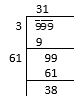The remainder is 38

So, the greatest 3 digit perfect square number is:

999 – 38 = 961

∴ 961 is the required greatest 3 digit perfect square number.

12. Find the smallest number which must be added to 2300 so that it becomes a perfect square.

Solution:

By using long division method let’s find the square root of 2300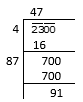The remainder is 91

Since, (47)2 < 2300

We take, the next perfect square number i.e., (48)2

(48)2 = 2304 > 2300

∴ The smallest number required to be added to 2300 to get a perfect square is

2304 – 2300 = 4

## RD Sharma Solutions for Class 8 Maths Exercise 3.5 Chapter 3 – Squares and Square Roots

Exercise 3.5 of RD Sharma Solutions for Chapter 3 Squares and Square Roots, this exercise primarily deals with the concepts related to the long division method, which provides the means for detecting the square root of a perfect square by the long division method.

The RD Sharma Solutions can help the students practice diligently while learning the fundamentals as it provides all the answers to the questions from the RD Sharma textbook. The solutions are solved in a precise manner which are easily understandable by the students to clear exams.